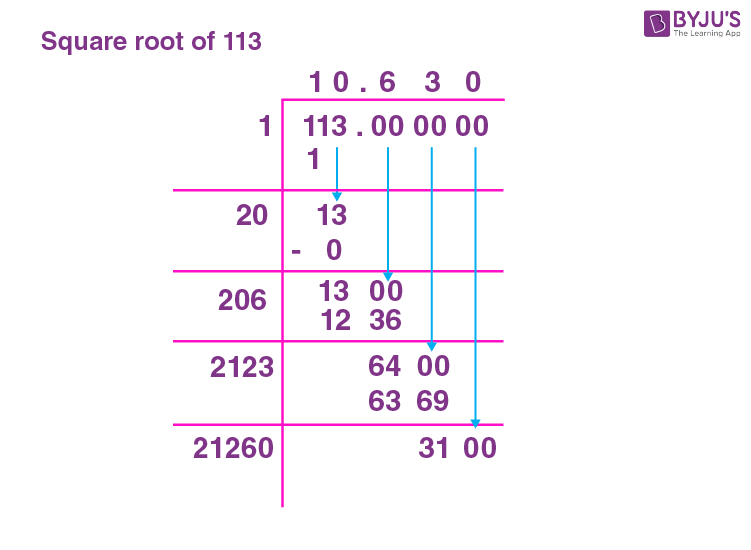# Square Root of 113

The square root of 113 is irrational. The approximate value of the square root of 113 is 10.63. When we say the square root of a number, it implies that there is a number which can be a positive or negative, that when multiplied by itself along with the same sign, the resulting number is the square number and the number that was multiplied by itself, it’s square root. Consider the number 10 × 10 = 100 and 11 × 11 = 121. 113 lies between 100 and 121. So the square root of 113 lies between 10 and 11 as 113 is not a perfect square number. Hence, the square root of 113 is also not a perfect integer. Therefore, √113 is an irrational number. You can refer to Finding Square roots.

Note the Following:

• The Square root of 113 = √113 where ‘√’ is the radical, and 113 is the radicand.
• Exponential Form of Square root of 113 = 1131/2
• Solution for √113 ≈ 10.63
• The square root of 113 is Irrational = True.

## What is the Square root of 113?

The square root of 113 is 10.63, as the square root of 113 is irrational.

 √113 = irrational =10.63

## How to Find the Square root of 113?

There are three methods to find the Square root of 113

• Prime Factorisation method
• Long Division method
• Repeated Subtraction method

### Square root of 113 by Prime Factorisation Method

In the prime factorization method, the steps are: Divide the given number with prime factors, group the same prime factors and then multiply the groups to find the square root of the given number.

The given number, 113 will be expressed as;

 113 113 × 1

113 = 113 × 1

113 is a prime number and it doesn’t have any other prime factors or factors. Hence it cannot be grouped and therefore it does not have a square root.

Therefore the square root of 113 = √113= 10.63

### Square root of 113 by Long Division Method

To understand the long division method, follow the below mentioned detailed steps;

Step 1:  Grouping the given number into pairs.

Step 2: Consider the first number, use the divisor such that the product is less than or equal to the first number. The divisors should be:

1 × 1 = 1

2 × 2 = 4

3 × 3 = 9

Step 3: Continue the division, by bringing the next pair

Step 4: The first divisor in the next division to be  (Divisor of first division + quotient of first division) such that;

d1 × 1 =

d2 × 2 =

d3 x 3 =

And so on.

Step 5: If the division is complete with the remainder as zero, then the quotient will be the square root. Else the number is not a perfect square number.

Applying the above steps to 113

Groups are 3 and 13

The squares are

1 × 1 = 1

2 × 2 = 4No further division is possible and the remainder is non-zero (13). Hence, 113 does not have a perfect square. Therefore 113 is an irrational number whose root lies between 10 and 11.

### Square root of 113 by Repeated Subtraction Method.

In the repeated Subtraction method, 113 is subtracted with odd numbers starting with 1 and the results with 3, 5, 7 and so on. If the result at any point becomes zero, then the number is a perfect square and the step at which it becomes zero is the square root.

The table below shows repeated subtraction for the given number 113.

 Step 1 113 – 1 = 112 Step 2 112 – 3 = 109 Step 3 109 – 5 = 104 Step 4 104 – 7 = 97 Step 5 97 – 9 = 88 Step 6 88 – 11 = 77 Step 7 77 – 13 = 64 Step 8 64 – 15 = 49 Step 9 49 – 17 = 32 Step 10 32 – 19 = 13 Step 11 13 – 21 = -8

The result at step 11 becomes negative and not zero (-8). Hence 113 is not a perfect square number. The square root of 113 lies between 10 and 11. – 8 indicates that 113 is short by 8 to become a perfect square number.

## Video Lessons

### Visualising square roots### Finding Square roots## Solved Examples

1. Which number is less than 113, that has a perfect square root?

100, which is less than 113 is a perfect square number. 10 is its perfect square root.

2. What is the square of 113?

113 square is 12769.

## Frequently Asked Questions on Square root of 113

### What is the Square root of 113?

The Square root of 113 is approximately 10.63.

### 113 is a perfect square number. True or False?

False. 113 is not a perfect square number.

### What are the prime factors of 113?

The prime factor of 113 is 113 only.

### What number should be added to 113 to make it a perfect square?

8 should be added to 113 to make it 121, which is a perfect square number.

### The square root of 113 is an irrational number. True or False?

True. The square root of 113 is an irrational number.